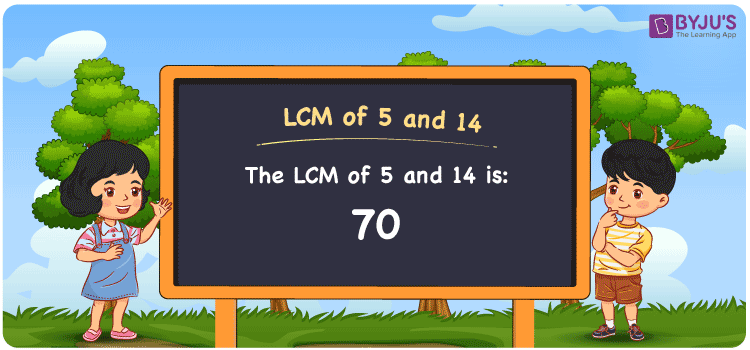# LCM of 5 and 14

LCM of 5 and 14 is 70. In Maths, the full form of LCM is the least common multiple. The LCM concept is mainly used to make the simplification process easier. The article Least Common Multiple (LCM) is designed by subject experts in an understandable language so that students grasp the LCM concept in an efficient manner. Students who get doubts while solving problems relying on the LCM concept are advised to follow this article to understand how the concept is solved on a daily basis. Let us learn how to calculate the least common multiple of 5 and 14 with solved examples and FAQs here.

## What is LCM of 5 and 14?

The answer to this question is 70.## How to Find LCM of 5 and 14?

The LCM of 5 and 14 can be determined by using the methods given below:

• Prime Factorisation
• Division method
• Listing the Multiples

### LCM of 5 and 14 Using Prime Factorisation Method

The prime factorisation method is one of the most common ways of calculating LCM. To find the LCM of 5 and 14, we use the steps as follows:

5 = 5

14 = 2 × 7

LCM (5, 14) = 2 × 5 × 7 = 70

### LCM of 5 and 14 Using Division Method

In the division method, to determine the least common multiple of 5 and 14, we divide the numbers 5 and 14 by their prime factors until we get the result as one in the complete row. The product of these divisors represents the least common multiple of 5 and 14.

 2 5 14 5 5 7 7 1 7 x 1 1

No further division can be done.

Hence, LCM (5, 14) = 2 × 5 × 7 = 70

### LCM of 5 and 14 Using Listing the Multiples

In this method, we list out the multiples of given numbers to find the least common multiple among them. The following are the multiples of 5 and 14.

Multiples of 5: 5, 10, 15, 20, 25, 30, 35, 40, 45, 50, 55, 60, 65, 70, ………….

Multiples of 14: 14, 28, 42, 56, 70, 84, 98, 112, 126, 140,………

LCM (5, 14) = 70

## Video Lesson on Applications of LCM## Solved Examples

1. What is the smallest number that is divisible by both 5 and 14?

Solution: 70 is the smallest number that is divisible by both 5 and 14.

2. What is the lowest common factor of 5 and 14?

Solution: The lowest common factor of 5 and 14 is 70.

## Frequently Asked Questions on LCM of 5 and 14

### What is the LCM of 5 and 14?

The LCM of 5 and 14 is 70.

### Is the LCM of 5 and 14 the same as the HCF of 5 and 14?

No. The LCM of 5 and 14 is 70 and the HCF of 5 and 14 is 1.

### 90 is the LCM of 5 and 14. True or False.

False. The LCM of 5 and 14 is 70.

### Calculate the GCF if the LCM of 5 and 14 is 70.

GCF × LCM = 5 × 14

Given

LCM = 70

GCF × 70 = 5 × 14

GCF = 1

### List the methods used to find the LCM of 5 and 14.

The methods used to find the LCM of 5 and 14 are

Prime Factorisation

Division Method

Listing the Multiples